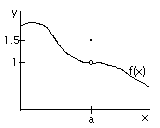1. ##Simple calculus question

lol, I haven't taken calc in years.

What's the answer to this question?

lim(x -> 0) [1 / x]

Is it +-Inf? +Inf? -Inf?Reply With Quote

2. ##Limit As x -> 0+ (from positive side) = +Inf

Limit As x-> 0- (from negative side) = -InfReply With Quote

3. ##The simple answer is that it doesn't exist since the limits as x approaches zero from the left side does not equal the limit as x approaches zero from the right side. If you graph it, you can see this much more easily.Edit: wait.... what the hell am I saying simple answer... it's the only answer 0.oReply With Quote

4. ##Strictly speaking, lim(x -> y) will never be equal for any limit as long the function's a "curve" (that is, f(x) = b, then lim(x -> y) = lim(x -> z). May be avoided by taking tops or bottoms of Sine or Cosine curves as well.)

But actually, the answer is Infinity. Cause ey, what's Infinity multiplied by -1?

Edit: Wait, is it undefined?Reply With Quote

5. ##...What? This doesn't make any sense. For one, what is lim(x -> y) not supposed to equal? You never say.

Negative infinity, which is a different number.(Unless you're in a special geometry, and we aren't.)

Long story short, the answer is DNE, which is standard shorthand for Does Not Exist. The limit approaches two different values from either side of the point you're considering, so essentially, it doesn't "approach" anything at all in the sense you want... hence, Does Not Exist. Watch Mean Girls if you want Lindsey Lohan to confirm it for you... in an overly excited, valley girl kind of way.EDIT: The answer isn't undefined. We say something is undefined when the question has been posed wrong... That's why 1/0 is undefined, because our system doesn't allow dividing by 0 anyway. This limit is a perfectly well-posed "question", it's just that the function in it happens to not be well-behaved at the point we're looking for.

That's why the term DNE exists. It's a fine point, though... The sort of thing math majors are interested in.Reply With Quote

6. ##Baww, bad example. I meant more like. Uh. Say we have a function f(x) = x. Then lim(x -> a) should be f(a + epsilon) = f(a - epsilon) where you let epsilon go towards 0. Then that will never happen unless f(x) = k or that f(x) = k * sin or k * cosin and that x is an element in the value handed to sin or cosin.

And bahh. Infinity isn't a number.Reply With Quote

7. ##Quite simply speaking, you can define the answer in terms of approach, or L'Hospital's rule. For general purposes and derivatives/integrals, if all approaches do not equal each other, the particular point in question is undefined. For example, tan(90 degrees) is undefined.

@ Morgana, I've only seen DNE in L'Hospital shyts, and nowhere else o.OReply With Quote

8. ##I'm positive the answer is DNE...if that's not confirmed yet.Reply With Quote

9. ##Fine, negative infinity is a different concept. Yeesh.

I don't understand your first point. You don't define what "k" is and I don't know how f(x) can equal a sine or cosine function when you already said f(x) = x. o.O And I don't get what you're trying to say with the trig anyway.

Does this help?In this case, the lim(x -> a) = 1, not 1.5. It doesn't matter what f(x) is at the point, it matters what it approaches.

I agree with you... the function tan(90 degrees) is undefined, since the function has no value at that point. But the limit of tan(x) as x approaches 90 degrees is DNE. Does that help?

Also, srsly guys, it's DNE. o.OReply With Quote

10. ##Uh. What? I may be missing the point of your post, but L'Hopital's rule isn't applicable in this scenario. It's DNE because of what shouri posted.Reply With Quote

11. ##Meh, I'm sorry. I meant f(x) = z, where z may be whatever you wish (ax + b, k and so forth)

k is a constant. Be it 1, 0, 90 or 1023. f(x) = x was just an example to see that f(a + epsilon) =/= f(a - epsilon), apart from when f(x) = k or when f(x) = k * (co)sin.Reply With Quote

12. ##Well, fk you morning, look what you made me type up D=
(I'm not a morning person, I am a fking zombie until 11 am)

Um yeah, 1/x is not an indeterminate form xD But I still stand by my reasoning that the limit as x approaches 0 DNE (what Fiel is asking for), but in most other context, the function is undefined at x = 0.Reply With Quote

13. ##The tangent thing you guys are talking about is similar to Fiel's question. tan(x) = sin(x)/cos(x), thus as x->90 degrees or (pi/2 rad), the function becomes undefined, thus the limit DNE.

I also have no flue what devil's getting at.Reply With Quote

14. ##Okay then, ignore me, lol.Reply With Quote

####Posting Permissions

• You may not post new threads
• You may not post replies
• You may not post attachments
• You may not edit your posts
•